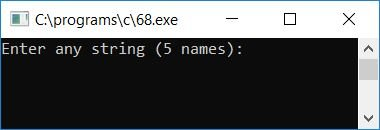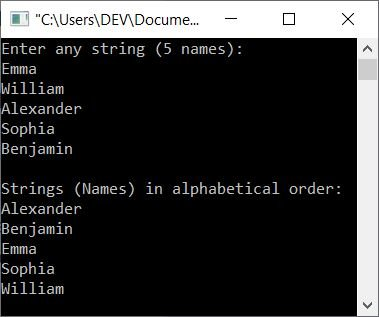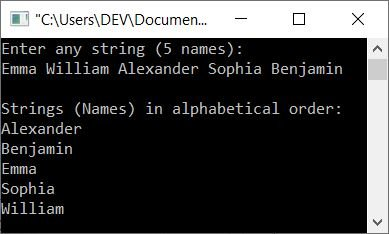# C Program to Sort Names in Alphabetical Order

In this tutorial, you will learn and get code for sorting given names by user (at run-time) in alphabetical order. For example, if the user provides the following five names as input:

• Emma
• William
• Alexander
• Sophia
• Benjamin

Then the output will be

• Alexander
• Benjamin
• Emma
• Sophia
• William

As you can see, all five names get arranged in alphabetical order (ascending). Let's move on and implement it in a C program.

```#include<stdio.h>
#include<conio.h>
#include<string.h>
int main()
{
char str, t;
int i, j;
printf("Enter any string (5 names):\n");
for(i=0; i<5; i++)
scanf("%s", str[i]);
for(i=1; i<5; i++)
{
for(j=1; j<5; j++)
{
if(strcmp(str[j-1], str[j])>0)
{
strcpy(t, str[j-1]);
strcpy(str[j-1], str[j]);
strcpy(str[j], t);
}
}
}
printf("\nStrings (Names) in alphabetical order:\n");
for(i=0; i<5; i++)
printf("%s\n", str[i]);
getch();
return 0;
}
```

The program was written in the Code::Blocks IDE; therefore, after a successful build and run, here is the sample run:Now supply any five names as a string and press the ENTER key to see the string with each word sorted in alphabetical order as shown here in the second snapshot.

You have to provide all five names one by one as a string. That is, enter the first name, say Emma, and hit the ENTER key; then enter the second name, say William, and hit the ENTER key; and so on.As the function used here to get the string input from the user is scanf(), you can also provide all 5 names as a string in one line, saying Emma William Alexander Sophia Benjamin. Here is the final snapshot of the sample run in this case:Here are some of the main steps used in the above program:

• Receive the list of 5 words as a string using a string array.
• Using a for loop, check the first character of all the words to compare and arrange in alphabetical order.
• That is, use the function strcmp() to compare the string. That is to check whether the first character of the first word is less than the first character of the second word or not.
• If it is, use the strcpy() function to reverse the word and continue to look for the next word within the same string.
• That is, again check whether the second word's first character is less than the third word's first character or not.
• If it is, reverse the word with each other using the strcpy() function, and so on.
• Now print the value of all five words as a string on the output.
• You will see that all five words are sorted or arranged in alphabetical order according to the first character of the word.

C Quiz

« Previous Program Next Program »